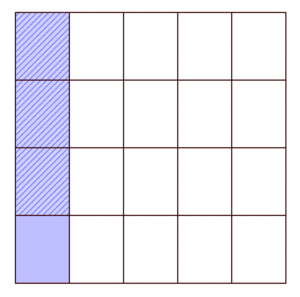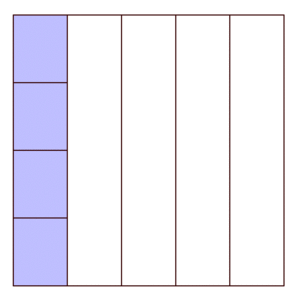# Connecting the Area Model to Context

Alignments to Content Standards: 5.NF.B.4.a

The diagram below represents one whole.Part One

Write a multiplication story that could be solved using the diagram with its two types of shading.  Explain how your story context relates to the diagram provided.

Part Two

Write the equation that represents this situation. Explain how your equation relates to the diagram provided.  Why is the diagram a good tool to use to solve this particular story context?

## IM Commentary

This task is designed to assess students’ conceptual understanding of the area model (one of the visual fraction models referred to in the standard for multiplying fractions).  If students are able to construct contexts that should use an area model, then the teacher will have information about the students’ readiness to move forward or need for more instruction.  It should be given after students have worked with the area model in class.  Students are asked to write a context that could be solved with the diagram provided.  Students must be able to interpret the diagram and then assign it a context that makes sense.  They have to identify the fractions being multiplied and then develop a word problem where the product of those two fractions represents the problem.  Then students are asked to write the equation that represents the situation and connect it back to the diagram.  The story context also should involve finding $\frac{3}{4}$ of $\frac{1}{5}$ of a quantity (some students might see this as $\frac{1}{4}$ of $\frac{1}{5}$ of a quantity, which is fine as long as they explain the correspondence between the problem and the diagram accurately).   The different aspects of this task challenge students to both decontextualize and contextualize the concept of multiplying fractions (Mathematical Practice #2).  By asking them to create the context rather than simply solve a given context, the task assesses different aspects of understanding.

This task was written as part of a collaborative project between Illustrative Mathematics, the Smarter Balanced Digital Library, and the Teaching Channel.

## Solution

Part One

Answers will vary from student to student.  However, the context must require the multiplication of $\frac{3}{4}$ and $\frac{1}{5}$. Here is a sample answer: Nan had a glass that contained $\frac{1}{5}$ liter of juice. She spilled $\frac{3}{4}$ of the juice in the glass. How many liters of juice did she spill?

Part Two

An equation is $\frac{3}{4}\times\frac{1}{5}$ = $\frac{3}{20}$ .  While multiplication is commutative, this equation is the best fit for the diagram and story based on the interpretation of as $\frac{3}{4}$ of $\frac{1}{5}$ as suggested by the diagram, which has $\frac{3}{4}$ of $\frac{1}{5}$ double shaded. In order to connect the equation to the diagram, students need to be able to show/explain where the two factors and the product are in the picture.  This can be achieved in many ways—here is one example: Start with $\frac{1}{5}$ of the square shaded—this represents $\frac{1}{5}$ liters of water. Then divide the $\frac{1}{5}$ into 4 equal pieces. Shade 3 of those pieces, which represents the $\frac{3}{4}$ of the amount of water she spilled. We can see that this represents $\frac{3}{20}$ of the entire rectangle by subdividing all of the fifths into 4 equal pieces so that all of the pieces are the same size. Because we found $\frac{3}{4}$ of $\frac{1}{5}$, we can represent it as  $\frac{3}{20}$. We can see in the picture that this is $\frac{3}{20}$.